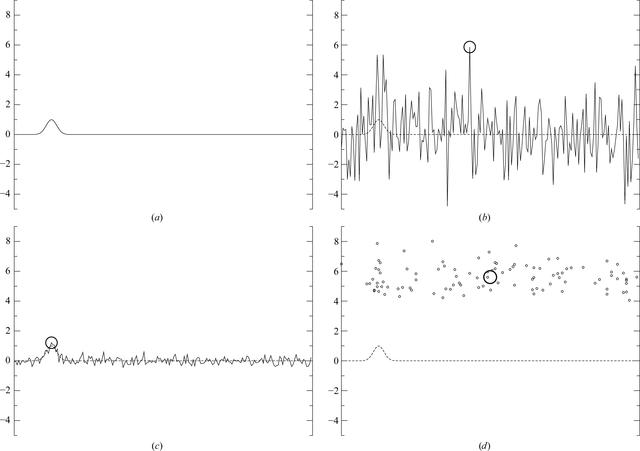disable zoom     view article Figure 1 A simulated example illustrating the issue of premature maximum picking. (a) A Gaussian representing the cross-correlation between the reference and the observation of a particle. (b) This cross-correlation distorted by Gaussian white noise of realistic intensity for the cross-correlation between a noise-free reference and one observed frame of one single particle (σ = 2; the circle indicates the maximum). (c) The average over 100 such noisy functions and its maximum. (d) The maxima of those 100 noisy functions (small circles) and their average (large circle). Note how the average of the noisy maxima (d) is much further from the true maximum than the maximum of the average (c). Our proposed method avoids picking individual maxima of noisy functions; it instead aims to maximize the cross-correlations of all particles and the prior smoothness assumptions simultaneously.

IUCrJ
ISSN: 2052-2525